# SAT II Math II : Solving Exponential, Logarithmic, and Radical Functions

## Example Questions

### Example Question #4 : Solving Functions

Rewrite as a single logarithmic expression: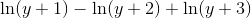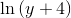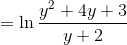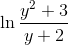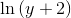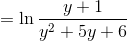Explanation:

Using the properties of logarithms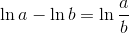and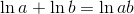,

simplify as follows: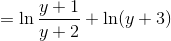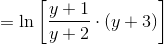### Example Question #5 : Solving Functions

Simplify by rationalizing the denominator: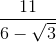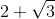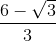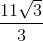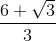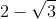Explanation:

Multiply the numerator and the denominator by the conjugate of the denominator, which is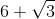. Then take advantage of the distributive properties and the difference of squares pattern: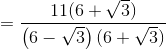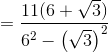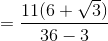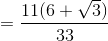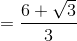### Example Question #6 : Solving Functions

Simplify: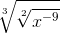You may assume thatis a nonnegative real number.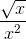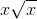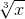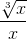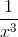Explanation:

The best way to simplify a radical within a radical is to rewrite each root as a fractional exponent, then convert back.

First, rewrite the roots as exponents.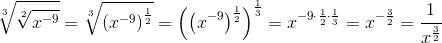Then convert back to a radical and rationalizing the denominator: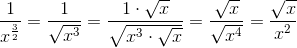### Example Question #7 : Solving Functions

Let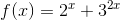.   What is the value of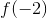?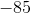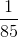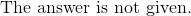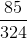Explanation:

Replace the integer as.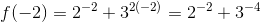Evaluate each negative exponent.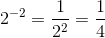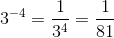Sum the fractions.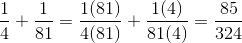The answer is:### Example Question #8 : Solving Functions

Find: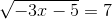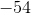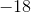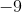Explanation:

Square both sides to eliminate the radical.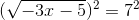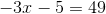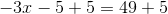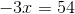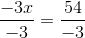The answer is: